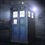# Probability doubt!

If one has to place 12 identical balls in 3 different boxes,is the number of ways $3^{12}$ or the number of non-negative integral solutions to the equation $a+b+c=12$?

I think it is the latter one,since if we are taking the product, we are assuming that the balls are distinct. Please provide insight.3 years, 9 months ago

This discussion board is a place to discuss our Daily Challenges and the math and science related to those challenges. Explanations are more than just a solution — they should explain the steps and thinking strategies that you used to obtain the solution. Comments should further the discussion of math and science.

When posting on Brilliant:

• Use the emojis to react to an explanation, whether you're congratulating a job well done , or just really confused .
• Ask specific questions about the challenge or the steps in somebody's explanation. Well-posed questions can add a lot to the discussion, but posting "I don't understand!" doesn't help anyone.
• Try to contribute something new to the discussion, whether it is an extension, generalization or other idea related to the challenge.

MarkdownAppears as
*italics* or _italics_ italics
**bold** or __bold__ bold
- bulleted- list
• bulleted
• list
1. numbered2. list
1. numbered
2. list
Note: you must add a full line of space before and after lists for them to show up correctly
paragraph 1paragraph 2

paragraph 1

paragraph 2

[example link](https://brilliant.org)example link
> This is a quote
This is a quote
    # I indented these lines
# 4 spaces, and now they show
# up as a code block.

print "hello world"
# I indented these lines
# 4 spaces, and now they show
# up as a code block.

print "hello world"
MathAppears as
Remember to wrap math in $$ ... $$ or $ ... $ to ensure proper formatting.
2 \times 3 $2 \times 3$
2^{34} $2^{34}$
a_{i-1} $a_{i-1}$
\frac{2}{3} $\frac{2}{3}$
\sqrt{2} $\sqrt{2}$
\sum_{i=1}^3 $\sum_{i=1}^3$
\sin \theta $\sin \theta$
\boxed{123} $\boxed{123}$

Sort by:

It also depends if the boxes can be empty or not

- 3 years, 9 months ago

They can be empty.

- 3 years, 9 months ago

If they can be empty then it has to be 91

- 3 years, 9 months ago

Yup!Actually in the book it was written as $3^{12}$,that is why I posted this note.Thanx!

- 3 years, 9 months ago

Sure. No problem

- 3 years, 9 months ago

Is it $66$?

- 3 years, 9 months ago

You are correct to think that it is the latter. Since the balls are identical, all that matters is the number of non-negative integer solutions $(a,b,c)$ to the equation you mention, the answer being $\binom{12 + 3 - 1}{3 - 1} = 91$.

If the balls are distinct and the order that they are placed in the boxes doesn't matter then the answer is $3^{12}$. If the order does matter, (e.g., if a solution where ball 1 is positioned under ball 2 is different than a solution with ball 2 positioned under ball 1), then things get more complicated.

- 3 years, 9 months ago

Thanks a lot sir!Actually this problem is from an exam(quite popular in India),and the options contained nothing like 91,so..

- 3 years, 9 months ago